Home > CC4 > Chapter A > Lesson A.1.9 > ProblemA-95

A-95.

For the following equations, solve for x. Check your solution, if possible. Record your work. Homework Help ✎

1. 3x − 7 = 3x + 1

2. −2x − 5 = −4x + 2

3. 2 + 3x = x + 2 + 2x

4. −(x − 2) = x + 2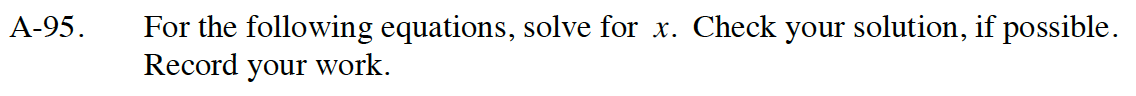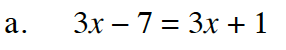Add 7 to both sides.

3x − 7 = 3x + 1
+7 +7

Subtract 3x from both sides.

3x = 3x + 8
−3x −3x

0 = 8

The statement is not true;
therefore, the answer is: No Solution.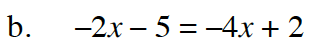Follow the steps in part (a).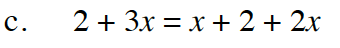Follow the steps in part (a).

Any number.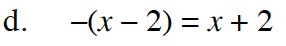How does the negative sign in front of the parentheses change what is inside?

Follow the steps in part (a).